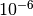# Trended Lomb-Scargle Periodogram¶

The standard Lomb-Scargle methods in `gatspy` (`LombScargle`, `LombScargleFast`, and `LombScargleAstroML`) fit a sinusoidal model to the data by minimizing the (weighted) squared residuals. These methods also support a floating mean term, which is an additional parameter that estimates the mean of the underlying model. The `TrendedLombScargle` class extends `LombScargle` by adding a trend parameter that is linear in time. Such a parameter may be appropriate when the underlying time series exhibits some non-stationarity.

## API of Trended Lomb-Scargle Model¶

The `TrendedLombScargle` class has the same API as `LombScargle`; the only difference is the underlying model that is fit to the data.

## Trended Lomb-Scargle Example¶

In order to motivate the trended Lomb-Scargle model, we’ll start with a one r-band RR Lyrae lightcurve and examine the effect of adding a linear trend on the estimated period. First, we’ll estimate the period as in Lomb-Scargle Periodogram before we add the trend:

```In : from gatspy import datasets, periodic

In : rrlyrae = datasets.fetch_rrlyrae()

In : lcid = rrlyrae.ids

In : t, mag, dmag, filts = rrlyrae.get_lightcurve(lcid)

In : mask = (filts == 'r')

In : model = periodic.LombScargleFast(fit_period=True)

In : model.optimizer.period_range = (0.2, 1.2)

In : model.fit(t_r, mag_r, dmag_r);

In : old_best_period = model.best_period

In : old_best_period
Out: 0.61431661211675215
```

The estimated period matches the period measured by Sesar 2010 to withindays.

Now we will add a small linear trend to the observed data and see how the estimated period is affected:

```In : slope = 0.005

In : mag_r += slope * (t_r - t_r)

In : model.fit(t_r, mag_r, dmag_r);

In : new_best_period = model.best_period

In : model.score([old_best_period, new_best_period])
Out: array([ 0.02881256,  0.87998659])
```

The addition of a small linear trend has greatly changed the estimated period; in fact, the old best period now has very little power in the estimated periodogram. Now let’s instead include a trend parameter by using the `TrendedLombScargle` model:

```In : tmodel = periodic.TrendedLombScargle(fit_period=True)

In : tmodel.optimizer.period_range = (0.2, 1.2)

In : tmodel.fit(t_r, mag_r, dmag_r);

In : trended_model_best_period = tmodel.best_period

In : trended_model_best_period
Out: 0.61431593030890863
```

The new trend parameter accounts for the linear increasing trend in the data, and we once again recover the original estimated period.